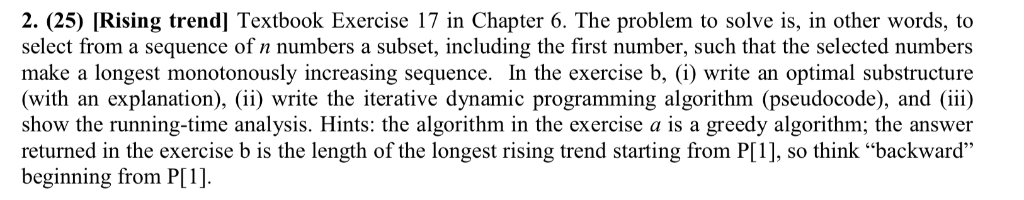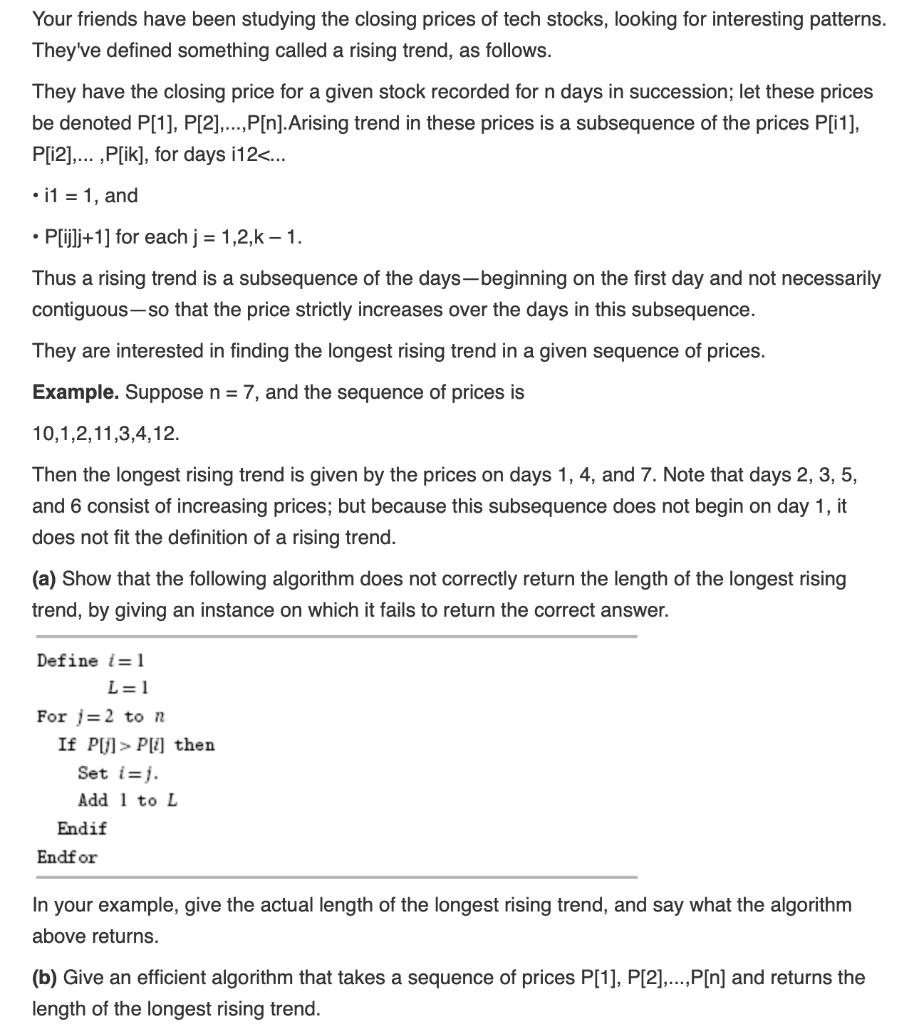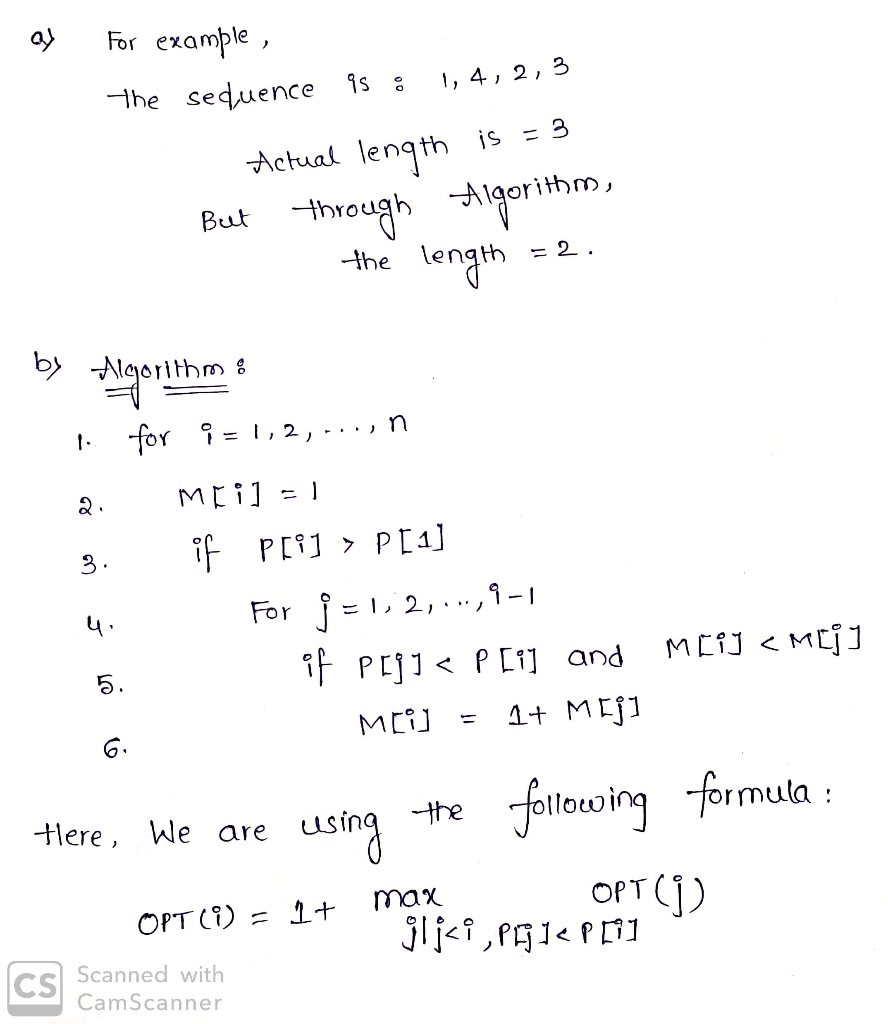# 2. (25) [Rising trend] Textbook Exercise 17 in Chapter 6. The problem to solve is, in other words...2. (25) [Rising trend] Textbook Exercise 17 in Chapter 6. The problem to solve is, in other words, to select from a sequence of n numbers a subset, including the first number, such that the selected numbers make a longest monotonously increasing sequence. In the exercise b, (i) write an optimal substructure (with an explanation),ii) write the iterative dynamic programming algorithm (pseudocode), and (iii) show the running-time analysis. Hints: the algorithm in the exercise a is a greedy algorithm; the answer returned in the exercise b is the length of the longest rising trend starting from P, so think "backward" beginning from P
Your friends have been studying the closing prices of tech stocks, looking for interesting patterns They've defined something called a rising trend, as follows They have the closing price for a given stock recorded for n days in succession; let these prices be denoted P, P,..,PIn].Arising trend in these prices is a subsequence of the prices P[i1], P[i2],... ,P[ik], for days i12 Pl] then Set i-j Add 1 to L Endif Endf or In your example, give the actual length of the longest rising trend, and say what the algorithm above returns (b) Give an efficient algorithm that takes a sequence of prices P, P,PIn] and returns the length of the longest rising trend##### Add Answer of: 2. (25) [Rising trend] Textbook Exercise 17 in Chapter 6. The problem to solve is, in other words...
More Homework Help Questions Additional questions in this topic.